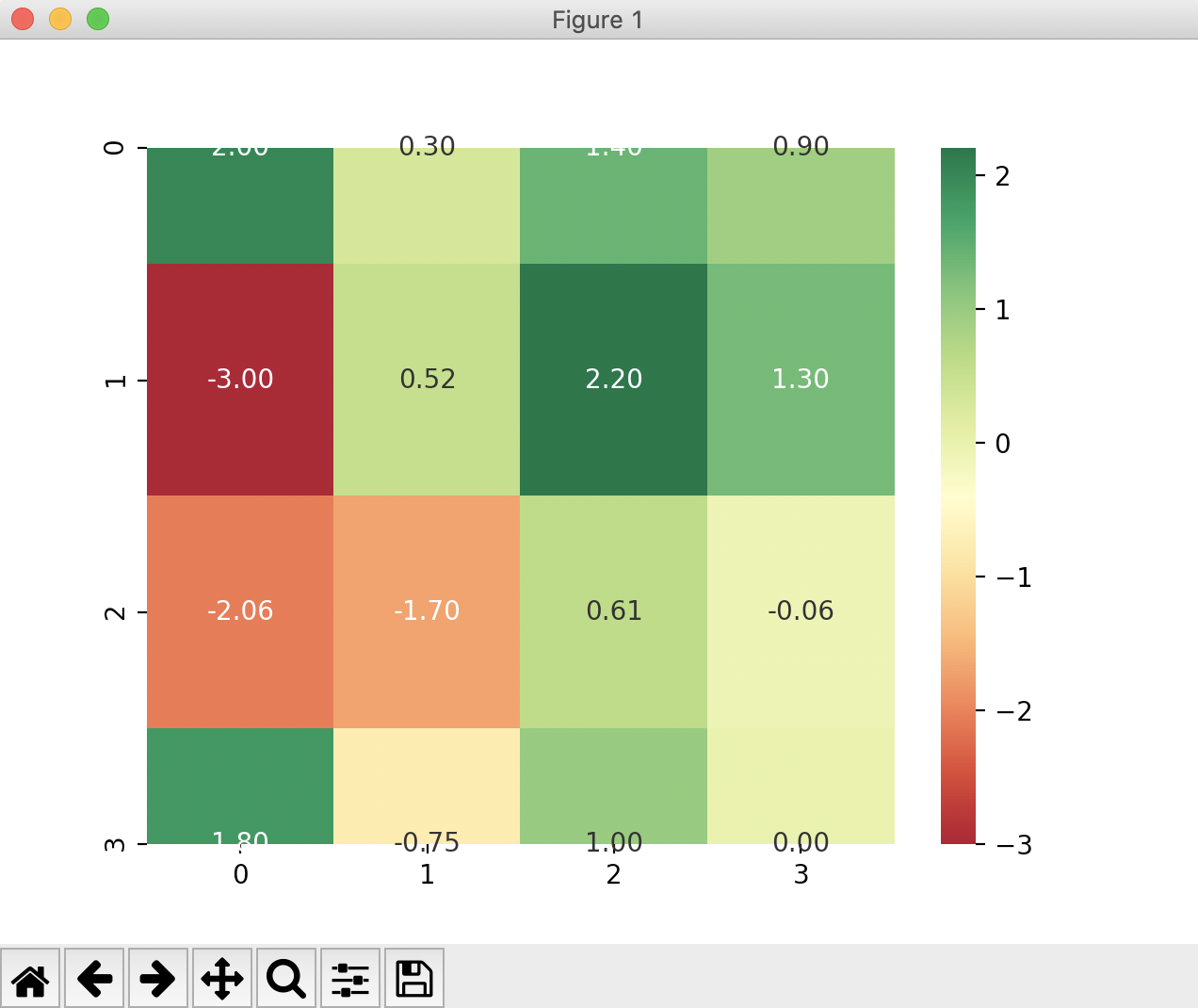# Drawing Heatmaps Using Seaborn

## Overview:

• A heatmap represents a matrix of information with transforming colors. There could be fewer to many colors typically from lighter ones to darker ones. The transformations and the intensity nature of the colors represent how the piece of information corresponding to the cell is changing in a specific time with respect to other cells.
• There are several scenarios where heatmaps come as a visual tool aiding in faster analysis. Price and volume movements of stocks from a major stock market index, COVID19 pandemic scenario across states are some of the examples.

## Example:

 # Example python program that draws a heatmap # of percentage price change for a set of stocks # using Python visulization library seaborn import matplotlib.pyplot as plt import seaborn as sbn import math import numpy as np # List of percentage changes l1 = [2.0, 0.3, 1.4, 0.9, -3.0, 0.52,       2.2, 1.3, -2.06, -1.7, 0.61, -0.06,       1.8, -0.75, 1.0, 0.0]; columns = 4; rows    = math.floor(len(l1)/columns); # Create a 2x2 ndarray grid         = np.ndarray((4, 4)); listIndex    = 0; # Fill in the 2x2 ndarray for i in range(0, rows):     for j in range(0, columns):         grid[i][j] = l1[listIndex];         listIndex = listIndex+1; print("Stock prices:"); print(grid); # Create a heatmap and display sbn.heatmap(grid, annot=True, fmt="f", cmap="RdYlGn"); plt.show();

## Output: